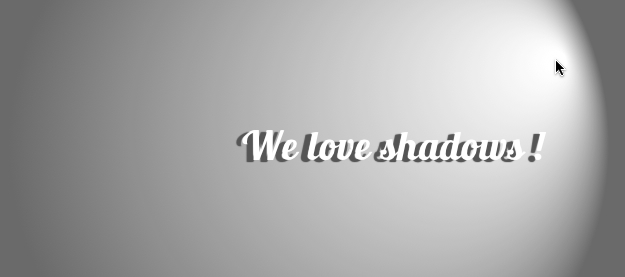# Flying memes

## We Love Shadows! A CSS3 and Javascript based light source

CSS3 has gradients and CSS3 has shadows, so why don’t try to use both to simulate a light source ? That is the basic idea behind this project. I’ve set-up a small demo which let you use the mouse to direct a light source over a text and observe its shadow change according to light position.

To simulate the light behavior I used a CSS3 gradient on the body background and a text-shadow property on the text. Both of these css3 attributes are then dynamically changed upon the user’s mouse move.

Here’s the snippet of the JQuery-flavored javascript I used:

``````\$(document).ready(function() {
\$('body').mousemove(function(event) {
\$('#instructions').hide();

// compute the center of the page and the distance between the center and the mouse
// coordinates
cx = Math.ceil(\$('body').width()  / 2);
cy = Math.ceil(\$('body').height() / 2);
dx = event.pageX - cx;
dy = event.pageY - cy;

// introducing a little parallax for the biggest light cone
x0 = Math.ceil(cx - (dx * 0.2));
y0 = Math.ceil(cy - (dy * 0.2));
x1 = event.pageX;
y1 = event.pageY;
r0 = 300;
r1 = 10;

// another parallax for the text shadow
sx = -dx * 0.03;
sy = -dy * 0.03;

// blur the shadow depending upon its distance
b  =  (Math.abs(sx) + Math.abs(sy)) * 0.2;

// simulate the light
\$('body').css('background-image', "-webkit-gradient(radial, " + x0 +" " + y0 +", "+ r0 +", "+ x1 +" "+ y1 +", "+ r1 +", from(#575757), to(#FFFFFF))");